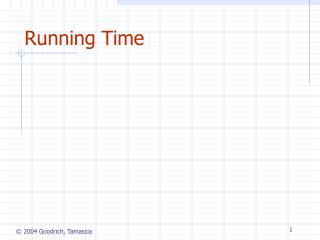DownloadDownload PresentationRunning Time

# Running Time

Télécharger la présentation## Running Time

- - - - - - - - - - - - - - - - - - - - - - - - - - - E N D - - - - - - - - - - - - - - - - - - - - - - - - - - -
##### Presentation Transcript

1. Running Time

2. Experimental Studies • Write a program implementing the algorithm • Run the program with inputs of varying size and composition • Use a method like System.currentTimeMillis() to get an accurate measure of the actual running time • Plot the results

3. Limitations of Experiments • It is necessary to implement the algorithm, which may be difficult • Results may not be indicative of the running time on other inputs not included in the experiment. • In order to compare two algorithms, the same hardware and software environments must be used

4. Theoretical Analysis • Uses a high-level description of the algorithm instead of an implementation • Characterizes running time as a function of the input size, n. • Takes into account all possible inputs • Allows us to evaluate the speed of an algorithm independent of the hardware/software environment

5. Example: find max element of an array AlgorithmarrayMax(A, n) Inputarray A of n integers Outputmaximum element of A currentMaxA fori1ton  1do ifA[i]  currentMaxthen currentMaxA[i] returncurrentMax Pseudocode • High-level description of an algorithm • More structured than English prose • Less detailed than a program • Preferred notation for describing algorithms • Hides program design issues

6. Control flow if…then… [else…] while…do… repeat…until… for…do… Indentation replaces braces Method declaration Algorithm method (arg [, arg…]) Input… Output… Method call var.method (arg [, arg…]) Return value returnexpression Expressions Assignment(like  in Java) Equality testing(like  in Java) n2 Superscripts and other mathematical formatting allowed Pseudocode Details

7. 2 1 0 The Random Access Machine (RAM) Model • A CPU • A potentially unbounded bank of memory cells, each of which can hold an arbitrary number or character Memory cells are numbered and accessing any cell in memory takes unit time.

8. Basic computations performed by an algorithm Identifiable in pseudocode Largely independent from the programming language Exact definition not important (we will see why later) Assumed to take a constant amount of time in the RAM model Examples: Evaluating an expression Assigning a value to a variable Indexing into an array Calling a method Returning from a method Primitive Operations

9. Seven Important Functions • Seven functions that often appear in algorithm analysis: • Constant  1 • Logarithmic  log n • Linear  n • N-Log-N  n log n • Quadratic  n2 • Cubic  n3 • Exponential  2n • In a log-log chart, the slope of the line corresponds to the growth rate of the function

10. Big-Oh Notation • Given functions f(n) and g(n), we say that f(n) is O(g(n))if there are positive constantsc and n0 such that f(n)cg(n) for n n0 • Example: 2n+10 is O(n) • 2n+10cn • (c 2) n  10 • n  10/(c 2) • Pick c = 3 and n0 = 10 Analysis of Algorithms

11. Big-Oh Example • Example: the function n2is not O(n) • n2cn • n c • The above inequality cannot be satisfied since c must be a constant Analysis of Algorithms

12. More Big-Oh Examples 7n-2 7n-2 is O(n) need c > 0 and n0 1 such that 7n-2  c•n for n  n0 this is true for c = 7 and n0 = 1 • 3n3 + 20n2 + 5 3n3 + 20n2 + 5 is O(n3) need c > 0 and n0 1 such that 3n3 + 20n2 + 5  c•n3 for n  n0 this is true for c = 4 and n0 = 21 • 3 log n + 5 3 log n + 5 is O(log n) need c > 0 and n0 1 such that 3 log n + 5  c•log n for n  n0 this is true for c = 8 and n0 = 2 Analysis of Algorithms

13. Relatives of Big-Oh • big-Omega • f(n) is (g(n)) if there is a constant c > 0 and an integer constant n0  1 such that f(n)  c•g(n) for n  n0 • big-Theta • f(n) is (g(n)) if there are constants c’ > 0 and c’’ > 0 and an integer constant n0  1 such that c’•g(n)  f(n)  c’’•g(n) for n  n0 Analysis of Algorithms

14. Intuition for Asymptotic Notation Big-Oh • f(n) is O(g(n)) if f(n) is asymptotically less than or equal to g(n) big-Omega • f(n) is (g(n)) if f(n) is asymptotically greater than or equal to g(n) big-Theta • f(n) is (g(n)) if f(n) is asymptotically equal to g(n) Analysis of Algorithms

15. Example Uses of the Relatives of Big-Oh • 5n2 is (n2) f(n) is (g(n)) if there is a constant c > 0 and an integer constant n0  1 such that f(n)  c•g(n) for n  n0 let c = 5 and n0 = 1 • 5n2 is (n) f(n) is (g(n)) if there is a constant c > 0 and an integer constant n0  1 such that f(n)  c•g(n) for n  n0 let c = 1 and n0 = 1 • 5n2 is (n2) f(n) is (g(n)) if it is (n2) and O(n2). We have already seen the former, for the latter recall that f(n) is O(g(n)) if there is a constant c > 0 and an integer constant n0  1 such that f(n) <c•g(n) for n  n0 Let c = 5 and n0 = 1 Analysis of Algorithms